# ML Aggarwal Class 7 ICSE Maths Model Question Paper 4

## ML Aggarwal Class 7 ICSE Maths Model Question Paper 4

(Based on Chapters 10 to 12)
Time allowed: 1 Hour
Maximum Marks: 25

General Instructions

• Questions 1-2 carry 1 mark each.
• Questions 3-5 carry 2 marks each.
• Questions 6-8 cany 3 marks each
• Questions 9-10 carry 4 marks each.

Choose the correct answer from the given four options (1-2):
Question 1.
In the given figure, if l || m then the value of x is
(a) 65°
(b) 105°
(c) 115°
(d) 125°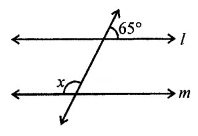Solution: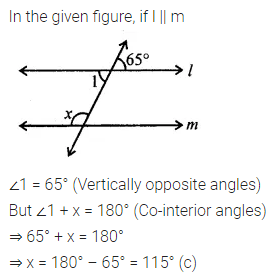Question 2.
A triangle whose two angles measure 40° and 100° is
(a) scalene
(b) isosceles
(c) equilateral
(d) right-angled
Solution:Question 3.
In the given figure, lines l and m intersect at O. If ∠1 + ∠3 = 222°, then find the measure of ∠2.Solution: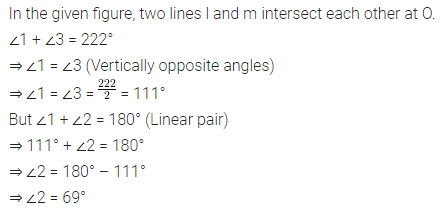Question 4.
In the given figure, AB = AC. Find the value of x.Solution: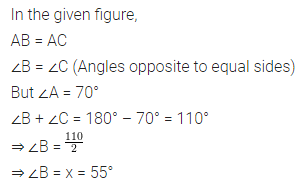Question 5.
If ∆PQR = ∆EFD, write the parts of ∆EFD that correspond to:
(i) ∠Q
(ii) $$\bar { PR }$$
(iii) ∠P
(iv) $$\bar { QR }$$
Solution: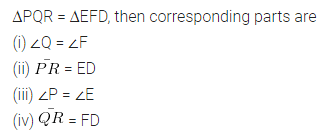Question 6.
In the given figure, l || m and p || q. Find the value of x, y, and z.Solution:Question 7.
In the given figure, AB = AC. Find the values of x and y.Solution: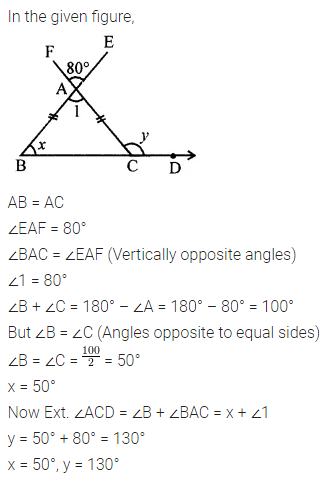Question 8.
If the lengths of two sides of a triangle are 5 cm and 7.5 cm then what can be the length of the third?
Solution: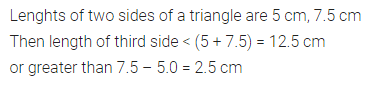Question 9.
An apple orchard is in the shape of a rectangle. If its length is 60 m and the length of one diagonal is 75 m, then find:
(i) the breadth of the orchard.
(ii) the perimeter of the orchard.
Solution:Question 10.
In the given figure, measures of some parts are given.
(i) State the three pairs of equal parts in ∆ABD and ∆ACD.
(ii) Is ∆ABD = ∆ACD? Give reason.
(iii) Is D mid-point of BC? Why?Solution: